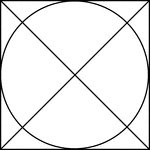### Square Circumscribed About A Circle

Illustration of a square, with diagonals drawn, circumscribed about a circle. This can also be described…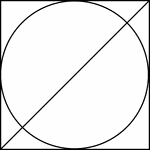### Square Circumscribed About A Circle

Illustration of a square, with 1 diagonals drawn, circumscribed about a circle. This can also be described…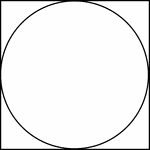### Square Circumscribed About A Circle

Illustration of a square circumscribed about a circle. This can also be described as a circle inscribed…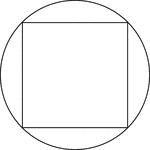### Square Inscribed In A Circle

Illustration of a square inscribed in a circle. This can also be described as a circle circumscribed…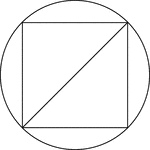### Square Inscribed In A Circle

Illustration of a square inscribed in a circle. This can also be described as a circle circumscribed…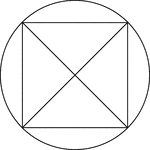### Square Inscribed In A Circle

Illustration of a square, with diagonals drawn, inscribed in a circle. This can also be described as…